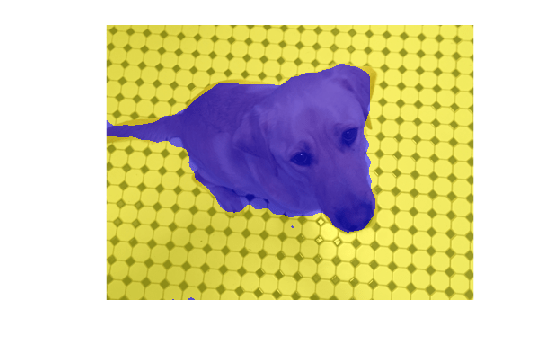# Texture Segmentation Using Gabor Filters

This example shows how to use texture segmentation to identify regions based on their texture. The goal is to segment the dog from the bathroom floor. The segmentation is visually obvious because of the difference in texture between the regular, periodic pattern of the bathroom floor, and the regular, smooth texture of the dog's fur.

From experimentation, it is known that Gabor filters are a reasonable model of simple cells in the Mammalian vision system. Because of this, Gabor filters are thought to be a good model of how humans distinguish texture, and are therefore a useful model to use when designing algorithms to recognize texture. This example uses the basic approach described in (A. K. Jain and F. Farrokhnia, "Unsupervised Texture Segmentation Using Gabor Filters",1991) to perform texture segmentation.

### Read and display input image

Read and display the input image. This example shrinks the image to make the example run more quickly.

```A = imread('kobi.png'); A = imresize(A,0.25); Agray = rgb2gray(A); figure imshow(A)```### Design Array of Gabor Filters

Design an array of Gabor Filters which are tuned to different frequencies and orientations. The set of frequencies and orientations is designed to localize different, roughly orthogonal, subsets of frequency and orientation information in the input image. Regularly sample orientations between [0,150] degrees in steps of 30 degrees. Sample wavelength in increasing powers of two starting from 4/sqrt(2) up to the hypotenuse length of the input image. These combinations of frequency and orientation are taken from [Jain,1991] cited in the introduction.

```imageSize = size(A); numRows = imageSize(1); numCols = imageSize(2); wavelengthMin = 4/sqrt(2); wavelengthMax = hypot(numRows,numCols); n = floor(log2(wavelengthMax/wavelengthMin)); wavelength = 2.^(0:(n-2)) * wavelengthMin; deltaTheta = 45; orientation = 0:deltaTheta:(180-deltaTheta); g = gabor(wavelength,orientation);```

Extract Gabor magnitude features from source image. When working with Gabor filters, it is common to work with the magnitude response of each filter. Gabor magnitude response is also sometimes referred to as "Gabor Energy". Each MxN Gabor magnitude output image in `gabormag(:,:,ind)` is the output of the corresponding Gabor filter `g(ind)`.

`gabormag = imgaborfilt(Agray,g);`

### Post-process the Gabor Magnitude Images into Gabor Features.

To use Gabor magnitude responses as features for use in classification, some post-processing is required. This post processing includes Gaussian smoothing, adding additional spatial information to the feature set, reshaping our feature set to the form expected by the `pca` and `kmeans` functions, and normalizing the feature information to a common variance and mean.

Each Gabor magnitude image contains some local variations, even within well segmented regions of constant texture. These local variations will throw off the segmentation. We can compensate for these variations using simple Gaussian low-pass filtering to smooth the Gabor magnitude information. We choose a sigma that is matched to the Gabor filter that extracted each feature. We introduce a smoothing term K that controls how much smoothing is applied to the Gabor magnitude responses.

```for i = 1:length(g) sigma = 0.5*g(i).Wavelength; K = 3; gabormag(:,:,i) = imgaussfilt(gabormag(:,:,i),K*sigma); end```

When constructing Gabor feature sets for classification, it is useful to add a map of spatial location information in both X and Y. This additional information allows the classifier to prefer groupings which are close together spatially.

```X = 1:numCols; Y = 1:numRows; [X,Y] = meshgrid(X,Y); featureSet = cat(3,gabormag,X); featureSet = cat(3,featureSet,Y);```

Reshape data into a matrix `X` of the form expected by the `kmeans` function. Each pixel in the image grid is a separate datapoint, and each plane in the variable `featureSet` is a separate feature. In this example, there is a separate feature for each filter in the Gabor filter bank, plus two additional features from the spatial information that was added in the previous step. In total, there are 24 Gabor features and 2 spatial features for each pixel in the input image.

```numPoints = numRows*numCols; X = reshape(featureSet,numRows*numCols,[]);```

Normalize the features to be zero mean, unit variance.

```X = bsxfun(@minus, X, mean(X)); X = bsxfun(@rdivide,X,std(X));```

Visualize the feature set. To get a sense of what the Gabor magnitude features look like, Principal Component Analysis can be used to move from a 26-D representation of each pixel in the input image into a 1-D intensity value for each pixel.

```coeff = pca(X); feature2DImage = reshape(X*coeff(:,1),numRows,numCols); figure imshow(feature2DImage,[])```It is apparent in this visualization that there is sufficient variance in the Gabor feature information to obtain a good segmentation for this image. The dog is very dark compared to the floor because of the texture differences between the dog and the floor.

### Classify Gabor Texture Features using kmeans

Repeat k-means clustering five times to avoid local minima when searching for means that minimize objective function. The only prior information assumed in this example is how many distinct regions of texture are present in the image being segmented. There are two distinct regions in this case. This part of the example requires the Statistics and Machine Learning Toolbox™.

`L = kmeans(X,2,'Replicates',5);`

Visualize segmentation using `label2rgb`.

```L = reshape(L,[numRows numCols]); figure imshow(label2rgb(L))```Visualize the segmented image using `imshowpair`. Examine the foreground and background images that result from the mask BW that is associated with the label matrix L.

```Aseg1 = zeros(size(A),'like',A); Aseg2 = zeros(size(A),'like',A); BW = L == 2; BW = repmat(BW,[1 1 3]); Aseg1(BW) = A(BW); Aseg2(~BW) = A(~BW); figure imshowpair(Aseg1,Aseg2,'montage');```